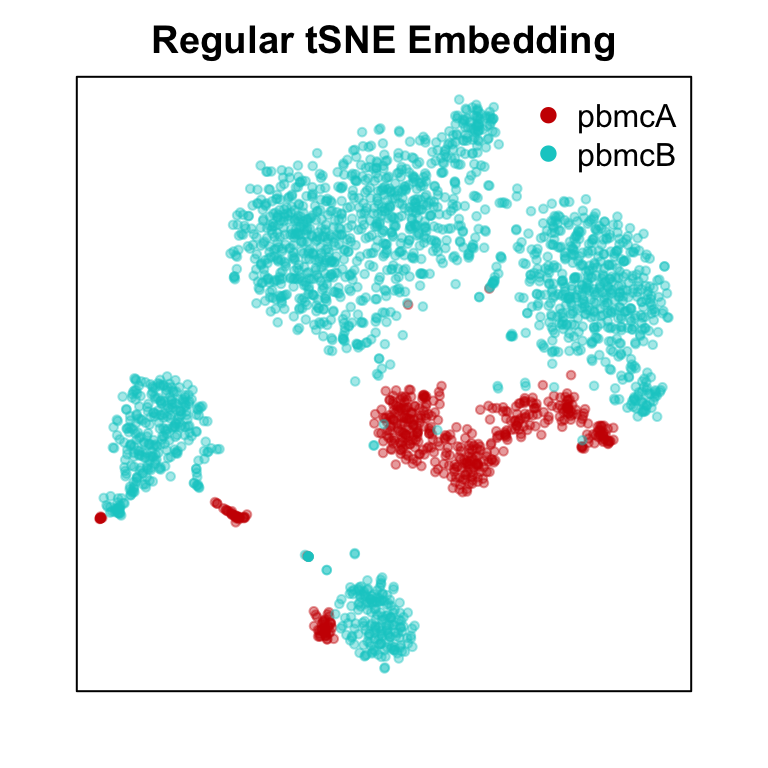# Contents

## 0.1 Introduction

`harmony` enables scalable integration of single-cell RNA-seq data for batch correction and meta analysis. In this tutorial, we will demonstrate the utility of `harmony` to jointly analyze single-cell RNA-seq PBMC datasets from two healthy individuals.

## 0.2 Installation

First, install `harmony` if you have not already done so.

``````if (!requireNamespace("BiocManager", quietly = TRUE))
install.packages("BiocManager")
BiocManager::install("harmony", version = "3.8")``````

Now we can load `harmony`

``library(harmony)``
``## Loading required package: Rcpp``

For this tutorial, we will use single-cell RNA-seq PBMC datasets that are readily available as part of the `MUDAN` package.

``install_github("JEFworks/MUDAN")``

Now we can load the data.

``library(MUDAN)``
``## Loading required package: Matrix``
``````data("pbmcA")
data("pbmcB")``````

For the purposes of a quick demonstration, we will downsize the number of cells in each PBMC dataset. To create a more challenging scenario, we will also make one dataset much smaller than the other.

``````# downsample
print(dim(pbmcA))
print(dim(pbmcB))
pbmcA <- pbmcA[, 1:500] # take 500 cells
pbmcB <- pbmcB[, 1:2000] # take 2000 cells``````
``````##  13939  2896
##  15325  7765``````

We can now combine the two datasets into one cell by gene counts matrix and use a `meta` vector to keep track of which cell belongs to which sample.

``````# combine into one counts matrix
genes.int <- intersect(rownames(pbmcA), rownames(pbmcB))
cd <- cbind(pbmcA[genes.int,], pbmcB[genes.int,])

# meta data
meta <- c(rep('pbmcA', ncol(pbmcA)), rep('pbmcB', ncol(pbmcB)))
names(meta) <- c(colnames(pbmcA), colnames(pbmcB))
meta <- factor(meta)

print(cd[1:5,1:2])
print(meta[1:5])``````
``````## 5 x 2 sparse Matrix of class "dgCMatrix"
##               frozen_pbmc_donor_a_AAACATTGCACTAG
## AL627309.1                                     .
## RP11-206L10.2                                  .
## RP11-206L10.9                                  .
## LINC00115                                      .
## NOC2L                                          .
##               frozen_pbmc_donor_a_AAACATTGGCTAAC
## AL627309.1                                     .
## RP11-206L10.2                                  .
## RP11-206L10.9                                  .
## LINC00115                                      .
## NOC2L                                          .
## frozen_pbmc_donor_a_AAACATTGCACTAG frozen_pbmc_donor_a_AAACATTGGCTAAC
##                              pbmcA                              pbmcA
## frozen_pbmc_donor_a_AAACATTGTAACCG frozen_pbmc_donor_a_AAACCGTGTGGTCA
##                              pbmcA                              pbmcA
## frozen_pbmc_donor_a_AAACCGTGTTACCT
##                              pbmcA
## Levels: pbmcA pbmcB``````

Given this counts matrix, we can normalize our data, derive principal components, and perform dimensionality reduction using tSNE. However, we see prominent separation by sample due to batch effects.

``````## CPM normalization
mat <- MUDAN::normalizeCounts(cd,
verbose=FALSE)
## variance normalize, identify overdispersed genes
matnorm.info <- MUDAN::normalizeVariance(mat,
details=TRUE,
verbose=FALSE)
## log transform
matnorm <- log10(matnorm.info\$mat+1)
## 30 PCs on overdispersed genes
pcs <- MUDAN::getPcs(matnorm[matnorm.info\$ods,],
nGenes=length(matnorm.info\$ods),
nPcs=30,
verbose=FALSE)

# TSNE embedding with regular PCs
emb <- Rtsne::Rtsne(pcs,
is_distance=FALSE,
perplexity=30,
verbose=FALSE)\$Y
rownames(emb) <- rownames(pcs)

# Plot
par(mfrow=c(1,1), mar=rep(2,4))
MUDAN::plotEmbedding(emb, groups=meta,
show.legend=TRUE, xlab=NA, ylab=NA,
main='Regular tSNE Embedding',
verbose=FALSE)``````Indeed, when we inspect certain cell-type specific marker genes (MS4A1/CD20 for B-cells, CD3E for T-cells, FCGR3A/CD16 for NK cells, macrophages, and monocytes, CD14 for dendritic cells, macrophages, and monocytes), we see that cells are separating by batch rather than by their expected cell-types.

``````par(mfrow=c(2,2), mar=rep(2,4))
invisible(lapply(c('MS4A1', 'CD3E', 'FCGR3A', 'CD14'), function(g) {
gexp <- log10(mat[g,]+1)
plotEmbedding(emb, col=gexp,
xlab=NA, ylab=NA, main=g,
verbose=FALSE)
}))``````